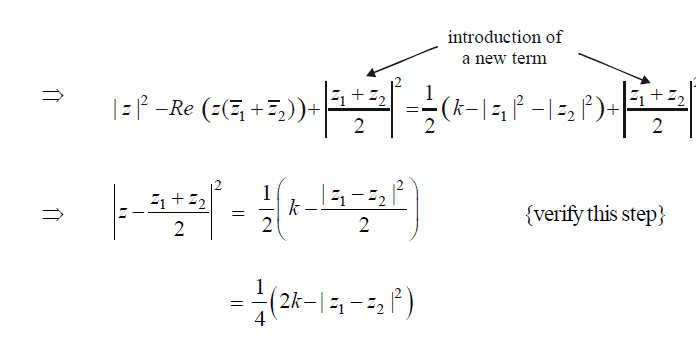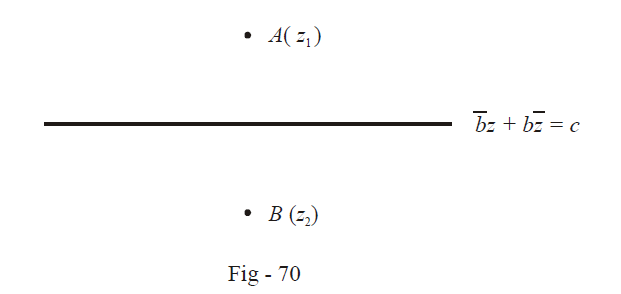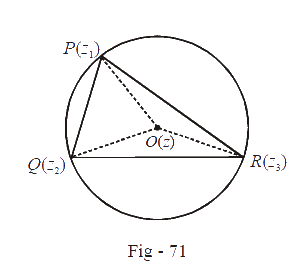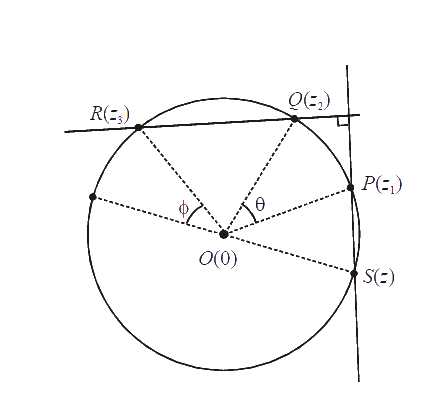# Problems On Doing Geometry With Complex Numbers

Example - 37

If $$z{_1}\text{ and}\;z{_2}$$  are fixed and $$z$$ satisfies

$|z - {z_1}{|^2} + |z - {z_2}{|^2} = k,$

find the possible values of  $$k$$ so that this equation represents a circle.

Solution: Let us try to reduce (simplify) this equation

\begin{align}&\qquad \;\;|z - {z_1}{|^2} + |z - {z_2}{|^2} = k\\ &\Rightarrow \quad 2|z{|^2} - 2{\mathop{\rm Re}\nolimits} (z{{\bar z}_1} + z{{\bar z}_2}) + |{z_1}{|^2} + |{z_2}{|^2} = k\\ &\Rightarrow \quad |z{|^2} - {\mathop{\rm Re}\nolimits} \left( {z({{\bar z}_1} + {{\bar z}_2})} \right) = \frac{1}{2}\left( {k - |{z_1}{|^2} - |{z_2}{|^2}} \right)\end{align}

Careful thinking will show that the left hand side can be transformed into a perfect square with the following manipulation.Thus, the centre is \begin{align}\,{z_0} = \frac{{{z_1} + {z_2}}}{2}\end{align} and the radius is \begin{align}\frac{1}{2}\sqrt {2k - |{z_1} - {z_2}{|^2}} .\end{align} For the radius to be defined the condition that $$k$$ must satisfy is

$k > \frac{1}{2}|{z_1} - {z_2}{|^2}$

These are the possible values of $$k.$$

Example - 38

Let $$\bar bz + b\bar z = c,\,\,b \ne 1$$ be a line in the complex plane. If a point $$z{_1}$$  is the reflection of a point $$z{_1}$$ through the line, show that $$c = {\bar z_1}b + {z_2}\bar b,$$

Solution:From the figure, it is obvious that the midpoint of $$AB$$ must lie on the given line, i.e, \begin{align}&\frac{{{z_1} + {z_2}}}{2}\end{align} should satisfy the equation of the line. Also, $$AB$$ must be perpendicular to the given line, i.e the complex slopes of $$AB$$ and the given line must add to 0.

\begin{align} &\Rightarrow \,\,\,\bar b\left( {\frac{{{z_1} + {z_2}}}{2}} \right) + b\left( {\frac{{{{\bar z}_1} + {{\bar z}_2}}}{2}} \right) = c\,\,\,\,\,\,\,...{\text{ }}\left( 1 \right) \\\\ &\text{and}\,\,\,\,\,\,\,\,\,\,\,\,\,\,\,\,\,\,\,\,\frac{{{z_1} - {z_2}}}{{{{\bar z}_1} - {{\bar z}_2}}} + \left( {\frac{{ - b}}{{\bar b}}} \right) = 0 \\\\&\Rightarrow \,\,\,\bar b\left( {{z_1} - {z_2}} \right) - b\left( {{{\bar z}_1} - {{\bar z}_2}} \right) = 0\,\,\,\,\,\,\,\,\,...{\text{ }}\left( 2 \right)\\\\& 2 \times \left( 1 \right) - \left( 2 \right)\,\,\,{\text{gives us the required relation.}}\\\end{align}

Example - 39

Find the circumcenter of the triangle whose vertices are given by the complex numbers $$z{_1}{\rm{ }},z{_2}{\rm{\;and\; }}z{_3}{\rm{ }}.$$

Solution:We have to find $$z,$$ the circumcenter $$O$$ of triangle $$PQR.$$ By virtue of being the circumcenter, $$z$$ is equidistant from $$z{_1}{\rm{ }},z{_2}{\rm{\;and\; }}z{_3}{\rm{ }}.$$ Therefore,

\begin{align}&\qquad\qquad\left| {z - {z_1}} \right| = \left| {z - {z_2}} \right| = \left| {z - {z_3}} \right|\\ &\Rightarrow \,\,\,(z - {z_1})(\bar z - {{\bar z}_1}) = (z - {z_2})(\bar z - {{\bar z}_2}) = (z - {z_3})(z - {{\bar z}_3})\\\,\,\,\,\,\,\,\,\,\,\,\,\,\,\,\,\,\,\,\,\,\,\,\,\,\,\,\,&\qquad\qquad\underbrace {\;\;\;\;\;\;\;\;\;\;\;\;\;\;\;\;\;\;\;}_{{\rm{Equality}}\,{\rm{A}}}\,\,\;\;\;\underbrace {\;\;\;\;\;\;\;\;\;\;\;\;\;\;\;\;\;\;\;}_{{\rm{Equality B}}}\\ \end{align}

$$\Rightarrow$$ From the first two terms in the equality above (Equality $$A$$) we get:

$\begin{gathered} z\, - z{{\bar z}_1} - {z_1}\bar z + {z_1}{{\bar z}_1} = z\, - z{{\bar z}_2} - {z_2}\bar z + {z_2}{{\bar z}_2} \hfill \\\Rightarrow \,\,\,\bar z({z_2} - {z_1}) = z({{\bar z}_1} - {{\bar z}_2}) + {\left| {{z_2}} \right|^2} - {\left| {{z_1}} \right|^2}\,\,\,\,\,\,\,\,\,\,\,\,\,\,\,\,\,\,...\left( 1 \right) \hfill \\\end{gathered}$

Similarly, from equality $$B,$$ we get

$\bar z({z_3} - {z_2}) = z({\bar z_2} - {\bar z_3}) + {\left| {{z_3}} \right|^2} - {\left| {{z_2}} \right|^2}\,\,\,\,\,\,\,\,\,...\left( 2 \right)$

Dividing (1) by (2), we get :

$\frac{{{z_2} - {z_1}}}{{{z_3} - {z_2}}} = \frac{{z({{\bar z}_1} - {{\bar z}_2}) + {{\left| {{z_2}} \right|}^2} - {{\left| {{z_1}} \right|}^2}}}{{z({{\bar z}_2} - {{\bar z}_3}) + {{\left| {{z_3}} \right|}^2} - {{\left| {{z_2}} \right|}^2}}}$

Solving for $$z,$$ we get

$z = \frac{{{{\left| {{z_1}} \right|}^2}({z_2} - {z_3}) + {{\left| {{z_2}} \right|}^2}({z_3} - {z_1}) + {{\left| {{z_3}} \right|}^2}({z_1} - {z_2})}}{{{{\bar z}_1}({z_2} - {z_3}) + {{\bar z}_2}({z_3} - {z_1}) + {{\bar z}_3}({z_1} - {z_2})}}$

Example - 40

$${z_1},\;{z_2}\;{\rm{and}}\;{z_3}$$ are three points on a circle centered at the origin. A point $$z$$ is chosen on the circle such that the line joining $$z$$ and $${z_1}$$ is perpendicular to the line joining $${z_2}$$ and $${z_3}$$ . Show that $$z{z_1} + {z_2}{z_3} = 0$$

Solution:We observe $$\theta = \phi$$ that, because of the perpendicularity of the two secants.

Now $$\angle \,SOR + \angle SOQ - \angle SOP = \pi$$ , (by using the fact that $$\theta = \phi$$ ). Therefore,

\begin{align}& \qquad\;\; \left( {\arg \left( {{z_3}} \right) - \arg (z)} \right) + \left( {\arg \left( {{z_2}} \right) - \arg (z)} \right) - \left( {\arg \left( {{z_1}} \right) - \arg (z)} \right) = \pi \\ &\Rightarrow \quad \arg \left( {{z_3}} \right) + \arg \left( {{z_2}} \right) = \pi + \arg \left( {{z_1}} \right) + \arg \left( z \right)\\ &\Rightarrow \quad \arg \left( {{z_2}{z_3}} \right) = \pi + \arg \left( {z{z_1}} \right)\\ &\Rightarrow \quad {z_2}{z_3} = - z{z_1} \Rightarrow \quad z{z_1} + {z_2}{z_3} = 0\end{align}

## TRY YOURSELF - IX

Q. 1 Find the real slopes of the following lines

(a) $$iz - i\bar z + 1 = 0$$

(b) $$\omega z + {\omega ^2}\bar z + 1 = 0$$

(c) $${e^{i\frac{\pi }{4}}}z + {e^{ + \frac{\pi }{4}}}\bar z = 0$$

(d) $$3z + 3\bar z + 2 = 0$$

Q. 2 Write the complex forms for the following lines

(a) $$x + y + 1 = 0$$

(b) $$\sqrt 3 x - y + 1 = 0$$

(c) $$x = 1$$ (d) y = 1

Q. 3 Consider a point  $$z{_0}$$ on the circle $$|z|\; = r$$ . What will be the equation of the tangent at $$z{_0}$$ ?

Learn from the best math teachers and top your exams

• Live one on one classroom and doubt clearing
• Practice worksheets in and after class for conceptual clarity
• Personalized curriculum to keep up with school